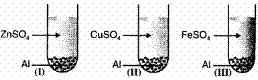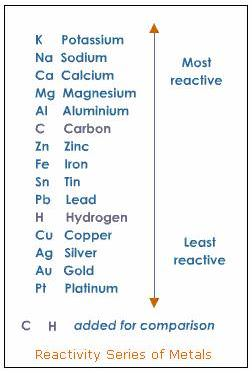### Chemical Reactions and Equations - Test Papers

CBSE Test Paper-01

Chapter 01 Chemical Reactions and Equations

1. Match the following with correct response. (1)

 (1) Combination reaction (A) ZnO+C→Zn+CO$ZnO+C\to Zn+CO$ (2) Decomposition reaction (B) 2H2O→2H2+O2$2{H}_{2}O\to 2{H}_{2}+{O}_{2}$ (3) Displacement reaction (C) 2Mg+O2→2MgO$2Mg+{O}_{2}\to 2MgO$ (4) Redox reaction (D) Fe+CuSO4→FeSO4+Cu$Fe+CuS{O}_{4}\to FeS{O}_{4}+Cu$
1. 1-D, 2-A, 3-C, 4-B
2. 1-C, 2-B, 3-D, 4-A
3. 1-B, 2-D, 3-A, 4-C
4. 1-A, 2-C, 3-B, 4-D
2. The chemical formula of lead sulphate is (1)

1. Pb2SO4
2. PbSO4
3. Pb2(SO4)3
4. Pb(SO4)2
3. The colour of zinc metal is (1)

1. Red dish brown
2. silvery
3. white
4. Grey
4. When you place an iron nail in copper sulphate solution, the reddish brown coating formed on the nail is (1)

1. smooth and shiny
2. rough and granule
3. soft and dull
4. hard and flaky
5.ObservationIIIIII
Solution after reactionColourlessColourlessColourless
Metal DepositedZnCuFe

Which of the following is correct conclusion? (1)

1. Al is more reactive than Cu and Fe but less reactive than Zn
2. Al is more reactive than Cu but less reactive than Zn and Fe
3. Al is more reactive than Zn and Cu but less reactive than Fe
4. Al is more reactive than Zn, Cu, Fe
6. Oil and fat containing food items are flushed with nitrogen. Why? (1)

7. What happens when copper powder is heated ? (1)

8. What is the principle of balancing of chemical equation? (1)

9. Why are food items packed in aluminium foils? (1)

10. Predict whether gold can displace copper from copper sulphate solution. (3)

11. What does one mean by exothermic and endothermic reactions ? Give examples. (3)

12. What are double displacement reaction? (3)

13. In the reaction PbS (s) + 4H2O2(aq) → PbSO4(s) + 4H2O (l), which is oxidizing agent and which is reducing agent? (3)

14. Write the formula and then balance the following equations. (5)

1. Butane (C4H10) + Oxygen $\to$ Carbon dioxide + Water
2. Magnesium + Silver nitrate $\to$ Magnesium nitrate + Silver
3. Lime water + Carbon dioxide $\to$ Calcium carbonate + Water
4. Sodium + Water $\to$ Sodium hydroxide + Hydrogen
5. Calcium carbonate + Water + Carbon dioxide $\to$ Calcium bicarbonate
15. There are different types of chemical reactions occurring around us or being carried out for the benefit of mankind, e.g. combination reactions, decomposition reactions, displacement reactions, precipitation reactions, reduction-oxidation (redox) reactions, photochemical reactions etc.
Now answer the following questions: (5)

1. Combustion of coke is a combination reaction. CO2 is not a pollutant. Then why is combustion of coke harmful?
2. Which reaction followed by two combination reactions are involved in white wash of walls?
3. Give one use of tin plating in daily life.
4. How photochemical reactions have played an important role in photography?

CBSE Test Paper-01
Chapter 01 Chemical Reactions and Equations

1. 1-C, 2-B, 3-D, 4-A
Explanation: 2 or more joining together to form single product is combination
Breaking of single reactant into 2 or more is decomposition
Both oxidation and reduction occurring simultaneously is redox reaction
More reactive metal displacing less reactive metal from its salt displacement reaction
1. PbSO4
Explanation: PbSO4
1. Grey
Explanation: It is whitish or bluish grey in colour.
1. soft and dull
Explanation: When you dip an iron nail in CuSO4, iron replaces copper from CuSO4, since it is more reactive than copper. The displaced copper gets deposited on the nail, which is soft and dull in nature.
Fe + CuSO4 $\to$ FeSO4 + Cu
1. Al is more reactive than Zn, Cu, Fe
Explanation: 2Al + 3ZnSO4 → Al2(SO4)3 + 3 Zn
2Al + 3CuSO4 → Al2(SO4)3 + 3 Cu
2Al + 3FeSO4 → Al2(SO4)3 + 3Fe
Al is more reactive than Zn, Cu and Fe because it displaces them from their salt solution.
1. In the presence of oxygen in the air, the fats present in the fatty food are oxidised to compounds which have a bad smell i.e. the food becomes rancid. Flushing with nitrogen cuts off the contact of food with oxygen and protects the food from rancidity.

2. Copper is oxidised to copper oxide.It means addition of oxygen.
Oxygen is reduced to copper oxide. It means addition of metal i.e reduction.
So, balanced chemical equation is: 2Cu + O₂→ 2CuO
A black colour copper (II) oxide is formed.

3. Chemical reactions follow the law of conservation of mass, meaning that the total mass of the reactants has to be equal to the total mass of the products. The number of each type of atom must also be conserved from reactants to products. Atoms cannot be created or destroyed, so if the equation cannot be balanced, there must be another reactant or product involved. Uncovering these missing materials can shed light on how the reactions take place, how to get a better yield of the products, or what waste products may be generated.

4. A protective coating of aluminium oxide (Al2O3) is formed on the surface of the foil which prevents it from getting corroded in the presence of air and water. Hence, food items are saved from getting spoilt when packed in aluminium foils.

5. Gold lies below copper in the activity series. This means that gold cannot displace copper from copper sulphate solution.6. Exothermic reaction: A chemical reaction in which heat is given out is known as exothermic reaction. For example
C(s) + O2 (g) → CO2(g) + Heat energy
NaOH (aq) + HCl(aq) → NaCl(aq) + H2O(l) + Heat energy

Endothermic reaction: A chemical reaction in which heat energy is absorbed is known as endothermic reaction. For example
N2(g) + O2(g) $\stackrel{Heat}{\to }$ 2NO(g)
C(s) + H2O(g) $\stackrel{Heat}{\to }$ CO(g) + H2(g)

7. Reactions in which ions are exchanged between two reactants forming new compounds are called double displacement reactions.
AB + CD $\to$ AC + BD
These are the reactions in which two different atoms or groups of atoms are displaced by another atoms/groups of atoms.
Example :

1. BaCl2(aq) + Na2SO4(aq) $\to$ BaSO4 (s)¯ + 2NaCl (aq)
2. AgNO3 (aq) + NaCl (aq) $\to$ AgCl(s)¯ + NaNO3 (aq)
Such reactions usually occur in ionic compound and are very fast reactions.
8. Oxidation - involves the loss of electrons or hydrogen OR gain of oxygen OR increase in oxidation state.
Reduction - involves the gain of electrons or hydrogen OR loss of oxygen OR decrease in oxidation state.
In the reaction PbS (s) + 4H2O2(aq) → PbSO4(s) + 4H2O (l), Our reactant is PbS, it takes up oxygen from H2O2 and changes to PbSO4 . It has undergone oxidation, or it has been oxidized.
H2O2 has lost oxygen and has changed to water, H2O. so it has been reduced.

9. The formula and balanced equations are:

1. Butane (C4H10) + Oxygen $\to$ Carbon dioxide + Water
2C4H10 + 13O$\to$ 8CO2 + 10H2O
2. Magnesium + Silver nitrate $\to$ Magnesium nitrate + Silver
Mg + 2AgNO3 $\to$ Mg(NO3)2 + 2Ag
3. Lime water + Carbon dioxide $\to$ Calcium carbonate + Water
Ca(OH)2 + CO2 $\to$ CaCO3 + H2O
4. Sodium + Water $\to$ Sodium hydroxide + Hydrogen
2Na + 2H2$\to$ 2NaOH + H2
5. Calcium carbonate + Water + Carbon dioxide $\to$ Calcium bicarbonate
CaCO3 + H2O + CO2 $\to$ Ca(HCO3)2
1. CO2 is not considered a pollutant up to a certain level. Rather, it helps in maintaining the temperature of the Earth. Combustion of coke is harmful as it increases the concentration of CO2 in the atmosphere which causes global warming due to the greenhouse effect.
2. The reaction of calcium with oxygen gives quicklime (CaO) which when mixed with water forms slaked lime Ca(OH)[combination reaction] and when slaked lime is applied on walls then it reacts with CO2 of the air to form CaCO3.
2Ca(s) + O2(g) $⟶$$\underset{\text{Quick lime}}{2CaO\left(s\right)}$
CaO(s) + H2O(l)$⟶$$\underset{\text{Slaked lime}}{Ca{\left(OH\right)}_{2}\left(aq\right)}$
Ca(OH)2(aq) + CO2(g) $⟶$$\underset{\text{Calcium carbonate}}{CaCO3\left(s\right)}$+ H2O(l)
3. Tin-plating is preferred in the tiffin boxes because tin is non-poisonous and hence, does not contaminate the food kept in them.
4. A photographic film used in black and white photography is a celluloid film coated with silver chloride. The silver chloride decomposes in the presence of sunlight and its working is totally based on this decomposition reaction giving silver which forms the image on the film.
$2AgCl\left(s\right)$ $\underset{\left(Decomposition\right)}{\overset{Sunlight}{\to }}$